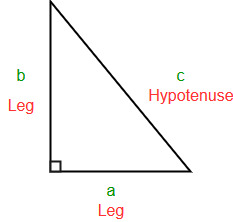# Find other two sides of a right angle triangle

• Difficulty Level : Hard
• Last Updated : 11 Jun, 2021

Given one side of right angle triangle, check if there exists a right angle triangle possible with any other two sides of the triangle. If possible print length of the other two sides.
Else print -1
Note: All the sides of triangle must be positive integers

Example 1:

Attention reader! All those who say programming isn't for kids, just haven't met the right mentors yet. Join the  Demo Class for First Step to Coding Coursespecifically designed for students of class 8 to 12.

The students will get to learn more about the world of programming in these free classes which will definitely help them in making a wise career choice in the future.

```Input : a = 3
Output : b = 4, c = 5
Explanation : a = 3, b = 4 and c = 5 form right
angle triangle because
32 + 42 = 9 + 16 = 25 = 52 => 32 + 42 = 52```

Example 2:

```Input : a = 11
Output : b = 60, c = 61
Explanation : a = 11, b = 60 and c = 61 form
right angle triangle because
112 + 602 = 121 + 3600 = 3721 = 612 => 112 + 602 = 612```

To solve this problem we first observe the Pythagoras equation. If a and b are the lengths of the legs of a right triangle and c is the length of the hypotenuse, then the sum of the squares of the lengths of the legs is equal to the square of the length of the hypotenuse.

This relationship is represented by the formula:

`a2 + b2 = c2`• Case 1 – a is an odd number: Given a, find b and c
```c2 - b2 = a2
OR
c = (a2 + 1)/2;
b = (a2 - 1)/2;```
• Above solution works only for case when a is odd, because a2 + 1 is divisible by 2 only for odd a.
• Case 2 – a is an even number: When c-b is 2 & c+b is (a2)/2
```c-b = 2 & c+b = (a2)/2
Hence,
c = (a2)/4 + 1;
b = (a2)/4 - 1;```

This works when a is even.

This solution doesn’t work for case of a = 1 and a = 2 because there isn’t any right angle triangle with side 1 or 2 with all integer sides.

## C++

 `// C++ program to print other two sides of right``// angle triangle given one side``#include ``using` `namespace` `std;` `// Finds two sides of a right angle triangle``// if it exist.``void` `printOtherSides(``int` `n)``{``    ``// if n is odd``    ``if` `(n & 1)``    ``{``        ``// case of n = 1 handled separately``        ``if` `(n == 1)``            ``cout << -1 << endl;``        ``else``        ``{``            ``int` `b = (n*n-1)/2;``            ``int` `c = (n*n+1)/2;``            ``cout << ``"b = "` `<< b``                 ``<< ``", c = "` `<< c << endl;``        ``}``    ``}``    ``else``    ``{``        ``// case of n = 2 handled separately``        ``if` `(n == 2)``            ``cout << -1 << endl;``        ``else``        ``{``            ``int` `b = n*n/4-1;``            ``int` `c = n*n/4+1;``            ``cout << ``"b = "` `<< b``                 ``<< ``", c = "` `<< c << endl;``        ``}``    ``}``}` `// Driver program to test above function``int` `main()``{``    ``int` `a = 3;``    ``printOtherSides(a);``    ``return` `0;``}`

## Java

 `// Java program to print other two``// sides of right angle triangle``// given one side` `class` `GFG``{``    ``// Finds two sides of a right angle``    ``// triangle if it they exist.``    ``static` `void` `printOtherSides(``int` `n)``    ``{``        ``// if n is odd``        ``if` `(n % ``2` `!= ``0``)``        ``{``            ``// case of n = 1 handled separately``            ``if` `(n == ``1``)``                ``System.out.println(``"-1"``);``            ``else``            ``{``                ``int` `b = (n * n -``1``) / ``2``;``                ``int` `c = (n *n  +``1``) / ``2``;``                ``System.out.println(``"b = "``+ b +``                                   ``", c = "``+c);``            ``}``        ``}``        ``else``        ``{``            ``// case of n = 2 handled separately``            ``if` `(n == ``2``)``                ``System.out.println(``"-1"``);``            ``else``            ``{``                ``int` `b = n * n / ``4` `- ``1``;``                ``int` `c = n * n / ``4` `+ ``1``;``                ``System.out.println(``"b = "``+ b +``                                   ``", c = "``+c);``            ``}``        ``}``    ``}``        ` `    ``// Driver code``    ``public` `static` `void` `main (String[] args)``    ``{``        ``int` `a = ``3``;``        ``printOtherSides(a);``    ``}``}` `// This code is contributed by Anant Agarwal.`

## Python

 `# Python program to print other``# two sides of right angle``# triangle given one side` `# Finds two sides of a right angle``# triangle if it they exist.``def` `printOtherSides(n):``    ` `    ``# if n is odd``    ``if``(n & ``1``):``        ` `        ``# case of n = 1 handled``        ``# separately``        ``if``(n ``=``=` `1``):``            ``print``(``-``1``)``        ``else``:``            ``b ``=` `(n ``*` `n ``-` `1``) ``/``/` `2``            ``c ``=` `(n ``*` `n ``+` `1``) ``/``/` `2``            ``print``(``"b ="``, b, ``", c ="``, c)``    ``else``:``        ` `        ``# case of n = 2 handled``        ``# separately``        ``if``(n ``=``=` `2``):``            ``print``(``-``1``)``        ``else``:``            ``b ``=` `n ``*` `n ``/``/` `4` `-` `1``            ``c ``=` `n ``*` `n ``/``/` `4` `+` `1``            ``print``(``"b ="``, b``", c ="``, c)` `# Driver Code``a ``=` `3``printOtherSides(a)` `# This code is contributed by``# Sanjit_Prasad`

## C#

 `// C# program to print other two``// sides of right angle triangle``// given one side``using` `System;` `class` `GFG {``    ` `    ``// Finds two sides of a right angle``    ``// triangle if it they exist.``    ``static` `void` `printOtherSides(``int` `n)``    ``{``        ` `        ``// if n is odd``        ``if` `(n % 2 != 0)``        ``{``            ` `            ``// case of n = 1 handled``            ``// separately``            ``if` `(n == 1)``            ``Console.WriteLine(``"-1"``);``            ``else``            ``{``                ``int` `b = (n * n - 1) / 2;``                ``int` `c = (n * n + 1) / 2;``                ``Console.Write(``"b = "``+ b +``                              ``", c = "``+ c);``            ``}``        ``}``        ``else``        ``{``            ` `            ``// case of n = 2 handled``            ``// separately``            ``if` `(n == 2)``            ``Console.Write(``"-1"``);``            ``else``            ``{``                ``int` `b = n * n / 4 - 1;``                ``int` `c = n * n / 4 + 1;``                ``Console.Write(``"b = "``+ b +``                              ``", c = "``+ c);``            ``}``        ``}``    ``}``        ` `    ``// Driver code``    ``public` `static` `void` `Main ()``    ``{``        ``int` `a = 3;``        ``printOtherSides(a);``    ``}``}` `// This code is contributed by Nitin Mittal.`

## PHP

 ``

## Javascript

 ``

Output:

`b = 4, c = 5`

This article is contributed by Pratik Chhajer . If you like GeeksforGeeks and would like to contribute, you can also write an article using write.geeksforgeeks.org or mail your article to review-team@geeksforgeeks.org. See your article appearing on the GeeksforGeeks main page and help other Geeks.